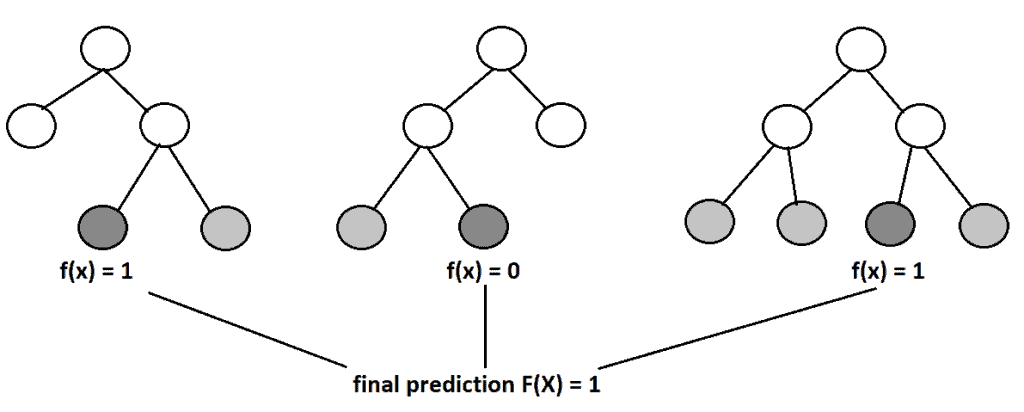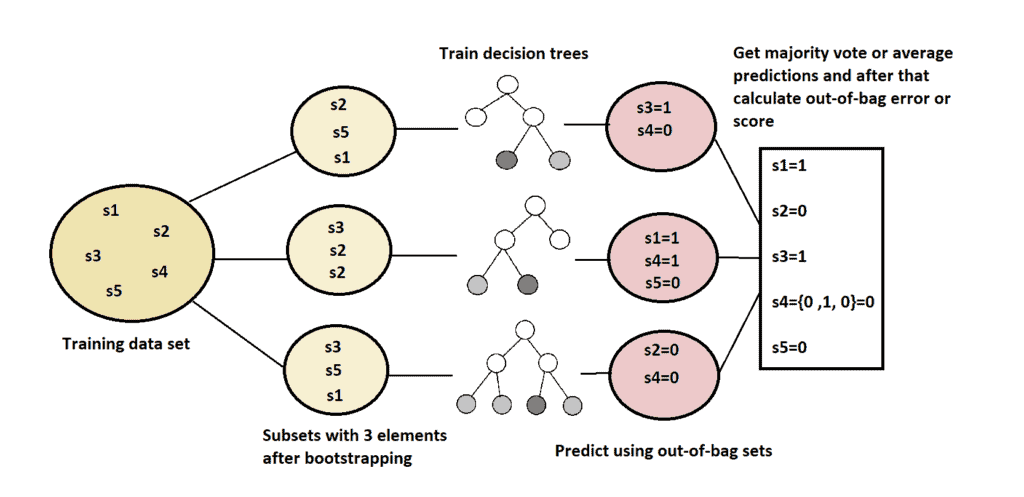## 1. Intro

In this tutorial, we’ll explore one of the most popular machine learning algorithms called random forest and the meaning of the out-of-bag error.

Besides that, we’ll briefly explain some of the terms related to random forests, such as decision tree, bagging, and bootstrapping.

## 2. Random Forest

Random forest is an ensemble learning method used for classification and regression. It relies on a set of decision trees to create a diverse prediction model. This algorithm is one of the most popular machine learning algorithms today because it offers robust accuracy, fast training time, and ease of interpretation.

In the first place, random forest uses the ensemble technique called bagging. Bagging is a technique for reducing variance when training a model on noisy datasets by creating multiple models so that each one compensates for the errors of the others. Basically, it means that a random forest is constructed of many decision trees, and the final prediction is calculated as the majority vote or average prediction of the trees:Random forest utilizes another compelling technique called bootstrapping. This is a statistical method that we can use to reduce the variance of machine learning algorithms. In short, it takes the original data set and creates a subset for each decision tree. Also, this method samples subsets randomly with the possibility of replacement. It means that we can select one sample or value multiple times.

Finally, to generalize the procedure, random forest limits the number of variables used for constructing decision trees. When we want to split the node of a decision tree, it considers only a subset of randomly selected variables (features). In the popular machine learning library called sci-kit-learn, this hyperparameter of random forest is known as “max_features”. Usually, the best practice is to set it to

•for classification
•for regression

whereis the total number of variables, andis the number of variables selected randomly for a particular node split.

## 3. Out-of-bag Error in Random Forests

Generally, in machine learning and data science, it is crucial to create a trustful system that will work well with the new, unseen data. Overall, there are a lot of different approaches and methods to achieve this generalization. Out-of-bag error is one of these methods for validating the machine learning model.

### 3.1. Definition

This approach utilizes the usage of bootstrapping in the random forest. Since the bootstrapping samples the data with the possibility of selecting one sample multiple times, it is very likely that we won’t select all the samples from the original data set. Therefore, one smart decision would be to exploit somehow these unselected samples, called out-of-bag samples.

Correspondingly, the error achieved on these samples is called out-of-bag error. What we can do is to use out-of-bag samples for each decision tree to measure its performance. This strategy provides reliable results in comparison to other validation techniques such as train-test split or cross-validation.

### 3.2. Probability of Out-of-bag Sample

Theoretically, with the quite big data set and the number of sampling, it is expected that out-of-bag error will be calculated on 36% of the training set. To prove this, consider that our training set hassamples. Then, the probability of selecting one particular sample from the training set is.

Similarly, the probability of not selecting one particular sample is. Since we select the bootstrap samples with replacement, the probability of one particular sample not being selectedtimes is equal to. Now, if the numberis pretty big or if it tends to infinity, we’ll get a limit below:

(1)### 3.3. Pseudocode

Accordingly, we can describe the whole procedure using pseudocode below:After all, more intuitively, we can present the whole process using the image below:## 4. Conclusion

In this article, we’ve described one efficient way of measuring error on the random forest model. The out-of-bag method is empirically tested in many papers, and it provides approximately the same model performance measurements as the test set. Moreover, this method might be even more convenient than simple train-test validation because we don’t need to divide our data into training and test.

This way, for smaller data sets, the machine learning algorithm will have more data for training.